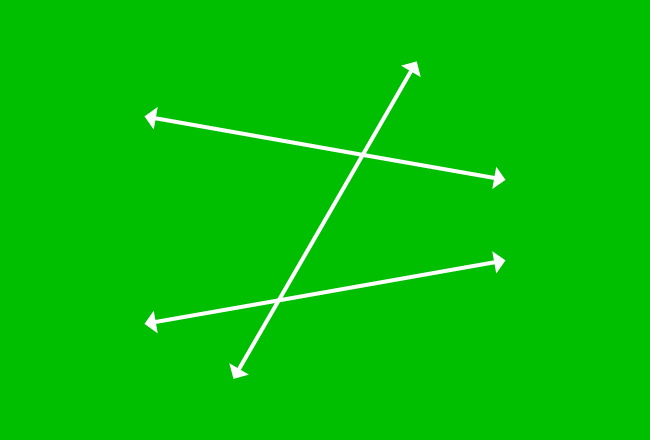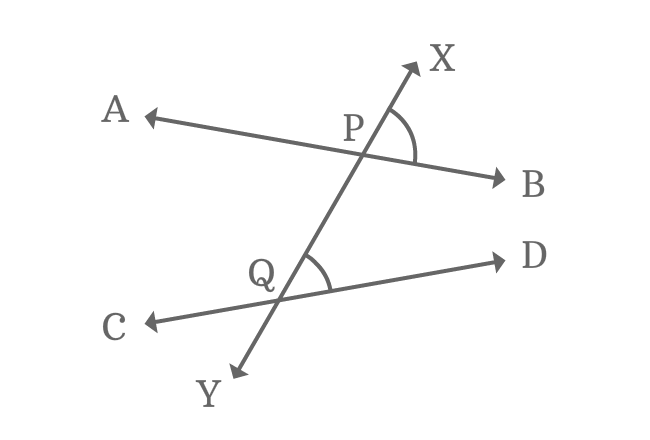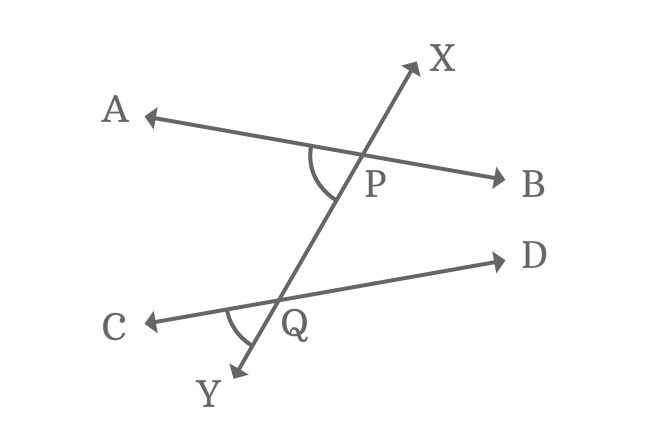# Corresponding Angles formed by Nonparallel Lines and their transversalFour pairs of angles have similar relative position at every intersection of every two straight lines when two nonparallel lines are intersected by their transversal. The angles are known as corresponding angles of the transversal of nonparallel lines.

Observe the picture, an angle has similarity in position with another angle and each pair of such angles are known as corresponding angles. Four pairs of corresponding angles are formed geometrically when a pair of nonparallel lines are cut by their transversal.

## Property

Geometrically, the corresponding angles which have similar relative position are not equal when two non-parallel lines are intersected by their transversal and this property can also be proved in geometric system.$\angle XPB$ and $\angle XQD$ are similar in relative position. So, they are corresponding angles but they both are not equal geometrically.

$\angle XPB \ne \angle XQD$

It is mainly due to the intersection of the two nonparallel lines by their transversal.

In the same way, the second set of corresponding angles $\angle YPB$ and $\angle YQD$ are also not equal.

$\angle YPB \ne \angle YQD$$\angle YQC$ and $\angle YPA$ are another set of corresponding angles and they are also not equal.

$\angle YQC \ne \angle YPA$

Similarly, the corresponding angles $\angle XQC$ and $\angle XPA$ are also not equal.

$\angle XQC \ne \angle XPA$

So, the animation tutorial is demonstrated that each pair of corresponding angles are not equal geometrically when two nonparallel lines are cut by their transversal.

Latest Math Topics
Jun 26, 2023
Jun 23, 2023

Latest Math Problems
Jul 01, 2023
Jun 25, 2023
###### Math Questions

The math problems with solutions to learn how to solve a problem.

Learn solutions

Practice now

###### Math Videos

The math videos tutorials with visual graphics to learn every concept.

Watch now

###### Subscribe us

Get the latest math updates from the Math Doubts by subscribing us.